# Archimedes' Principle, Buoyancy Experiments and Flotation Force

Eugene is a qualified control/instrumentation engineer Bsc (Eng) and has worked as a developer of electronics & software for SCADA systems.

## Who Was Archimedes?

Archimedes of Syracuse was a Greek astronomer, scientist and mathematician who was born circa 287 BC. Amongst his many works as a great scientist of the classical period was laying the ground work for modern calculus as well as proving geometric theorems, working out approximations for pi and calculating the surface area and volumes of 3D solids.

## What is Archimedes' Principle?

Archimedes' principle states that the upthrust or buoyant force on an object in a fluid is equal to the weight of the displaced fluid. Displaced means pushed out of the way, so for instance when you drop stones into a container of water, you displace the water and it rises in the container. A force can be though of as a push or pull. The fluid doesn't have to be water, it can be any other liquid or gas, e.g. air. For more detailed information on forces, see my physics tutorial:

Types of Forces Explained: Physics for Kids

## Experiments to Understand the Archimedean Principle

Let's do some experiments to investigate and understand the principle of Archimedes.

## Experiment 1. Investigating Negative Buoyancy

### Step 1. Weigh the Object

Imagine we have an object suspended from a weighing scales. For instance it could be an iron weight like the one in the diagram below. We're going to lower it into a tank of water filled to the brim, level with the overflow outlet. The weight may float or it may become submerged, but it doesn't matter and doesn't affect our experiment. Before we lower it into the tank, the weighing scales tells us that its weight is 6 kg.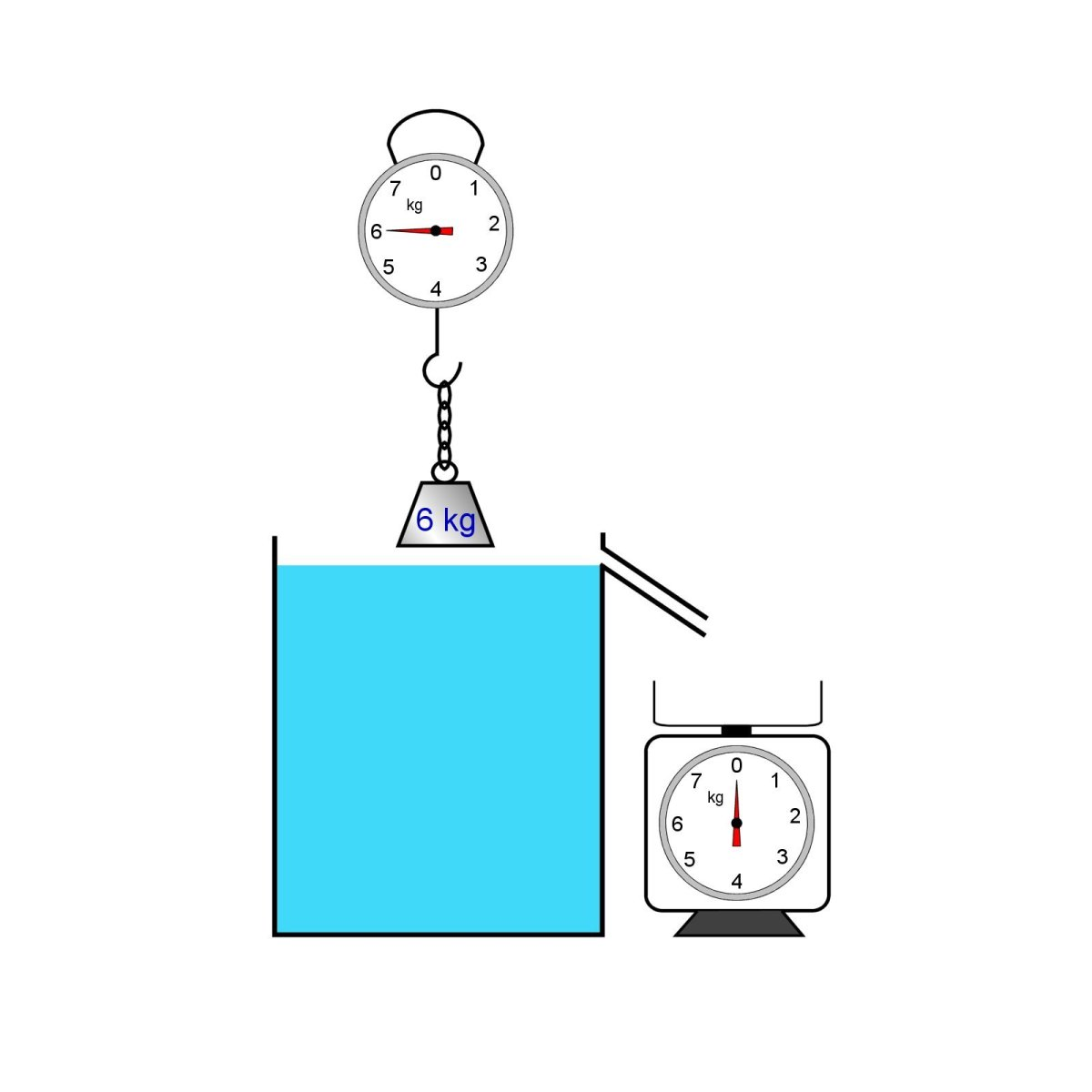Experiment to investigate the principle of Archimedes.© Eugene Brennan

### Step 2. Weigh the Displaced Water

As the weight is lowered, water is displaced and overflows into the pan on the second scales. When the weight is fully submerged we find that the water we collected weighs 2 kg.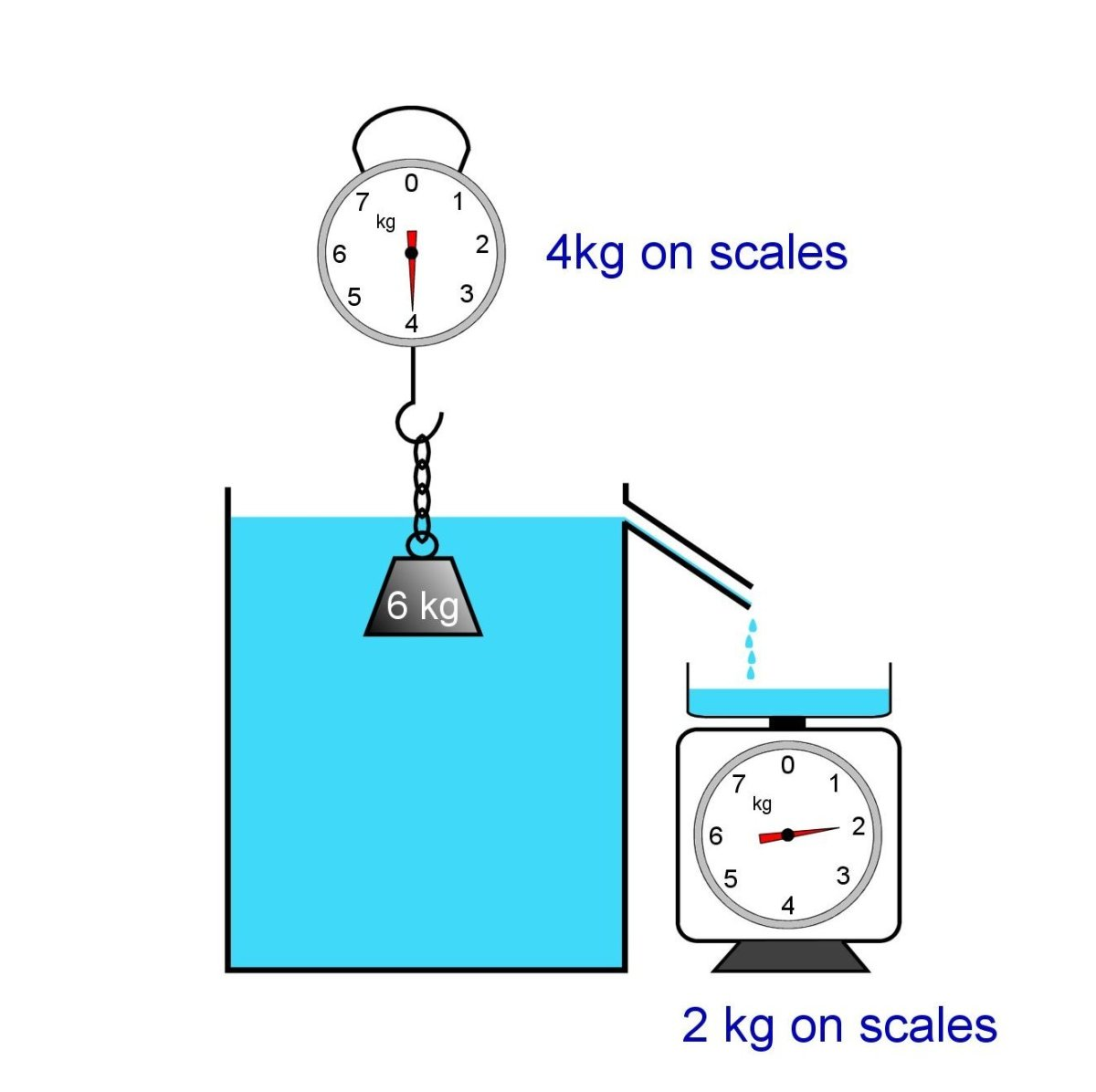Demonstrating the Archimede's principle. Weight submerged in water. Displaced water is weighed.© Eugene Brennan

### Step 3. Check the Weight on the First Scales

We now check the weight on the first scales again.

We find that the weight indicated is only 4 kg this time.

### Step 4. Do Some Calculations

We discover that when we subtract the new measurement of the iron weight indicated on the scales from the previous measurement, it tallies with the weight we measured on the second scales.

So 6 kg - 4 kg = 2 kg

## Principle of Archimedes

We've just discovered Archimedes' principle!

"The upthrust on a body submerged or floating in a fluid equals the weight of the fluid displaced"

Scroll to Continue

## Read More From Owlcation

How come the weight indicated on the first scales is now less than it was before?

It's because of the upthrust or buoyancy force.

This accounts for the difference and the object appearing lighter.

The 6 kg weight acts downwards, but it's as though 2 kg is pushing upwards acting as support and lessening the weight of the iron. So the scales indicates a smaller net weight of 4 kg. This upthrust equals the weight of the displaced water we collected in the pan of the second scales.

However, the mass of the object is still the same = 6 kg.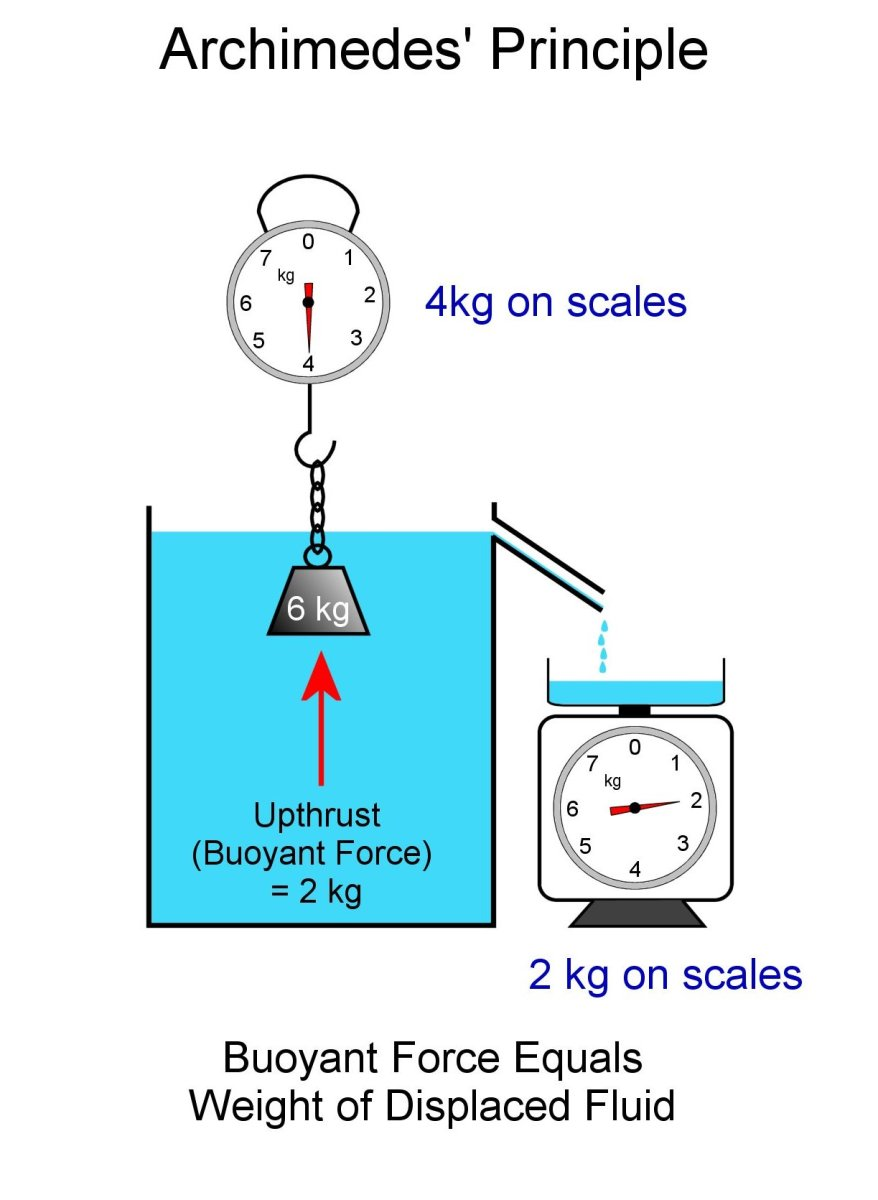The principle of Archimedes. Buoyant force equals the weight of the displaced liquid.© Eugene Brennan

## What are the 3 Types of Buoyancy?

### Negative, Positive and Neutral Buoyancy

An object placed in a fluid such as water can do three things:

1. It can sink. We call this negative buoyancy.
2. It can float. We call this positive buoyancy. If we push the object below the surface of the water and let go, the positive buoyancy force pushes it back up again above the surface.
3. It can stay submerged below the surface and neither sink to the bottom nor float back to the surface. If it's moved to a different depth below the surface, it stays in that position. This is called neutral buoyancy.

## Negative Buoyancy and Sinking Bodies

In the first experiment we did earlier, the iron weight sank below the water as it was lowered. The 6 kg iron weight weight we used displaces water. However the weight of the water displaced is only 2 kg. So the buoyant force is 2 kg acting upwards on the iron weight. Since this is less than 6 kg, it isn't enough to support the weight in the water. We call this negative buoyancy. If the weight was detached from the hook of the weighing scales, it would sink.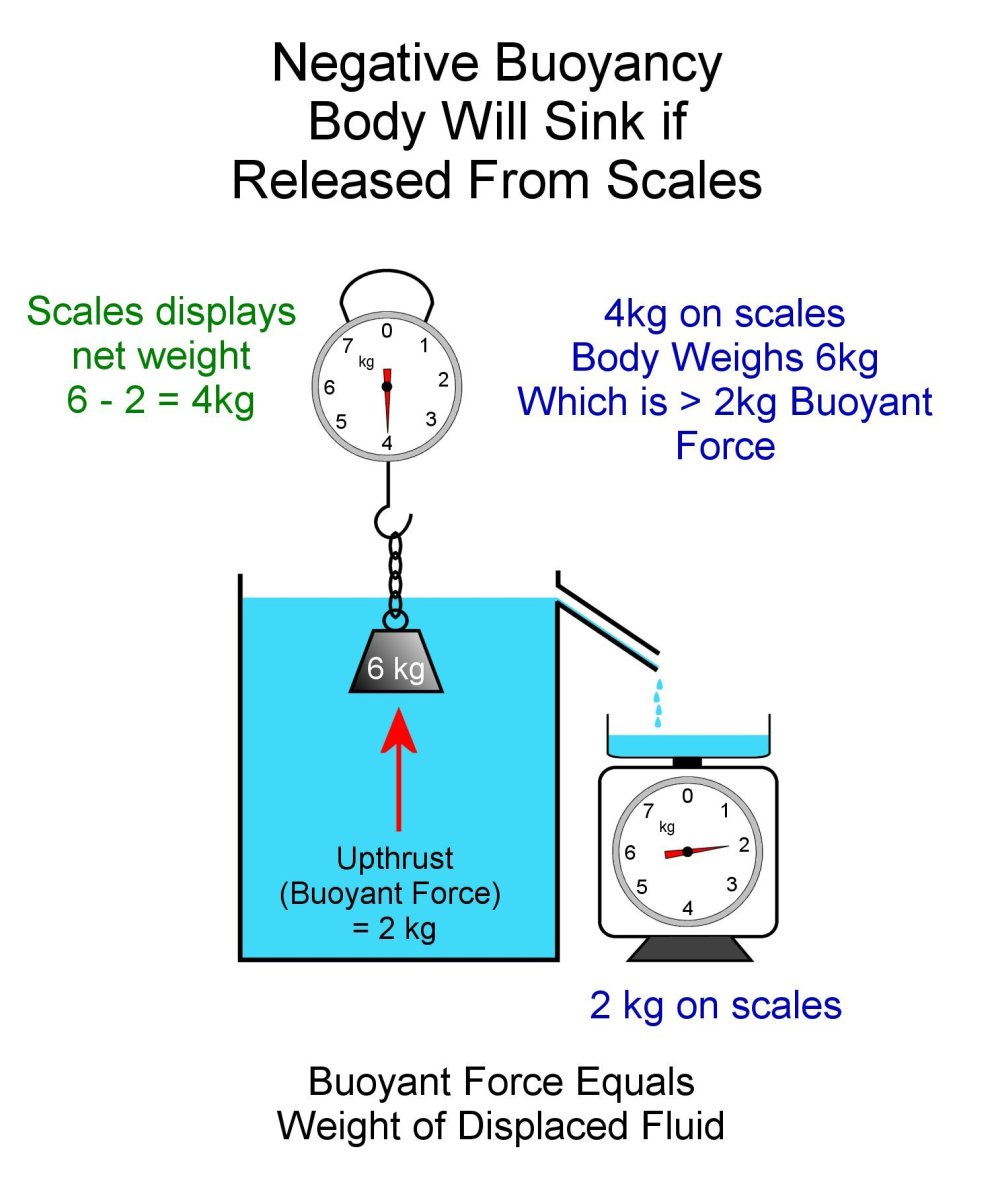Negative buoyancy. Buoyant force is less than the weight of the submerged body.© Eugene Brennan

## What are Examples of Things That Need Negative Buoyancy?

• Anchors need to have negative buoyancy so they can sink to the ocean floor.
• Fishing net sinkers to keep nets openAn anchor on a shipAnalogicus via Pixabay.com

## Experiment 2. Investigating Positive Buoyancy

This time we lower a hollow steel ball onto the surface.

## Positive Buoyancy and Floating Objects

What happens if a weight floats and doesn't sink? In the diagram below we lower a hollow steel ball into the tank. This time we know the weight is 3 kg. The chain goes slack if we move the scales closer to the water surface, because the weight floats and doesn't pull down on it. (In reality the chain has weight and will pull down on the scales, but for the sake of the experiment, let's imagine it's weightless.) The scale indicates 0 kg. The water displaced weighs the same as the weight this time.

So what happens in this scenario is that the ball displaces water and settles lower and lower in the water until the upthrust equals its weight. The force of gravity on the object acting downwards, i.e. its weight, is balanced by a buoyant force or upthrust acting upwards. Since the two are the same, the object floats.

In this second scenario, the object doesn't become fully submerged.

If we push the ball below the surface, it will displace more water, increasing the buoyant force. This force will be greater than the weight of the ball and the positive buoyancy will cause it to rise up out of the water and just displace enough water until the buoyant force and weight are equal again.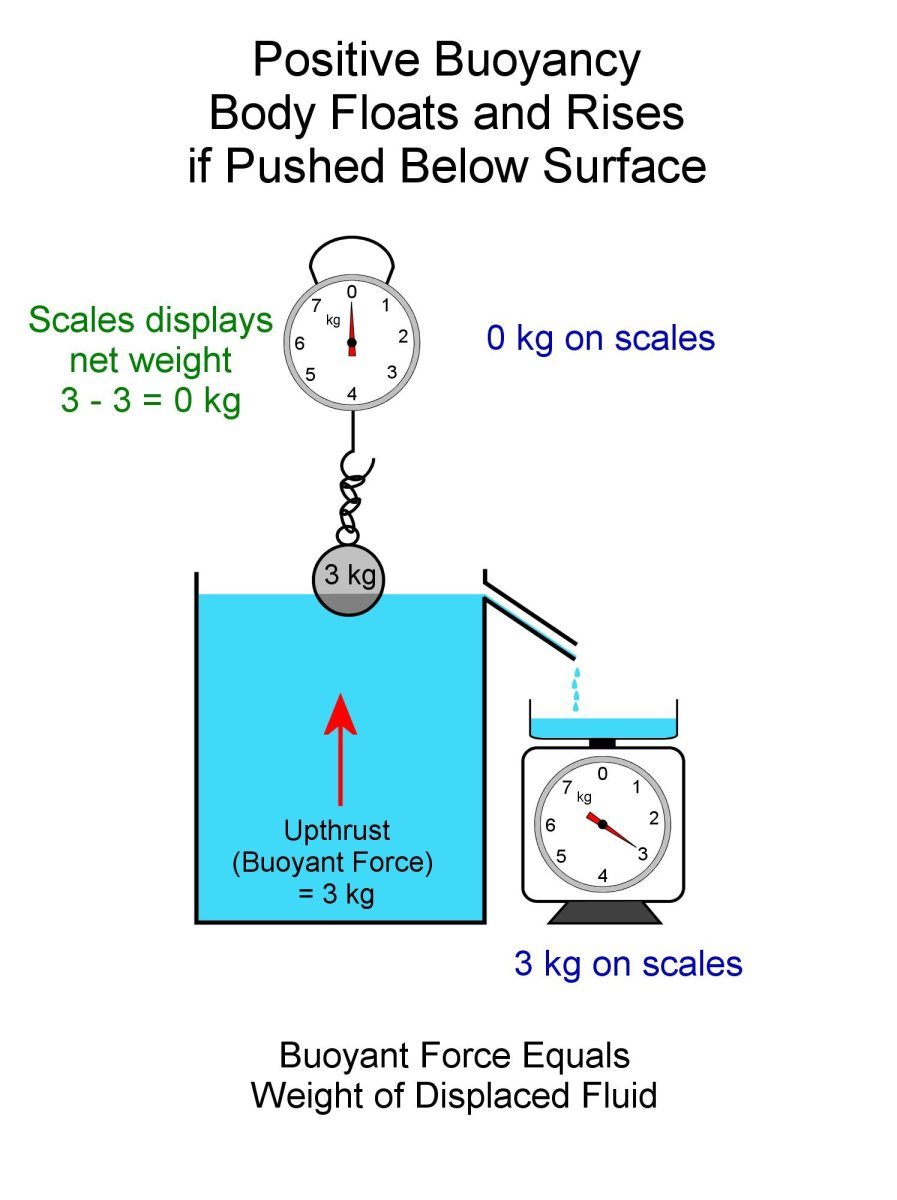Positive buoyancy. The buoyant force and weight of the hollow steel ball are equal.© Eugene Brennan

## What are Examples of Things That Need Positive Buoyancy?

• Lifebelts (lifebuoys)
• Marking and meteorological buoys
• Ships
• Swimmers
• Life jackets
• Floats on fishing lines
• Floats in toilet cisterns and float switches
• Flotation tanks/bags for recovering lost cargo/archaeological artefacts/submerged vessels
• Floating oil rigs and wind turbines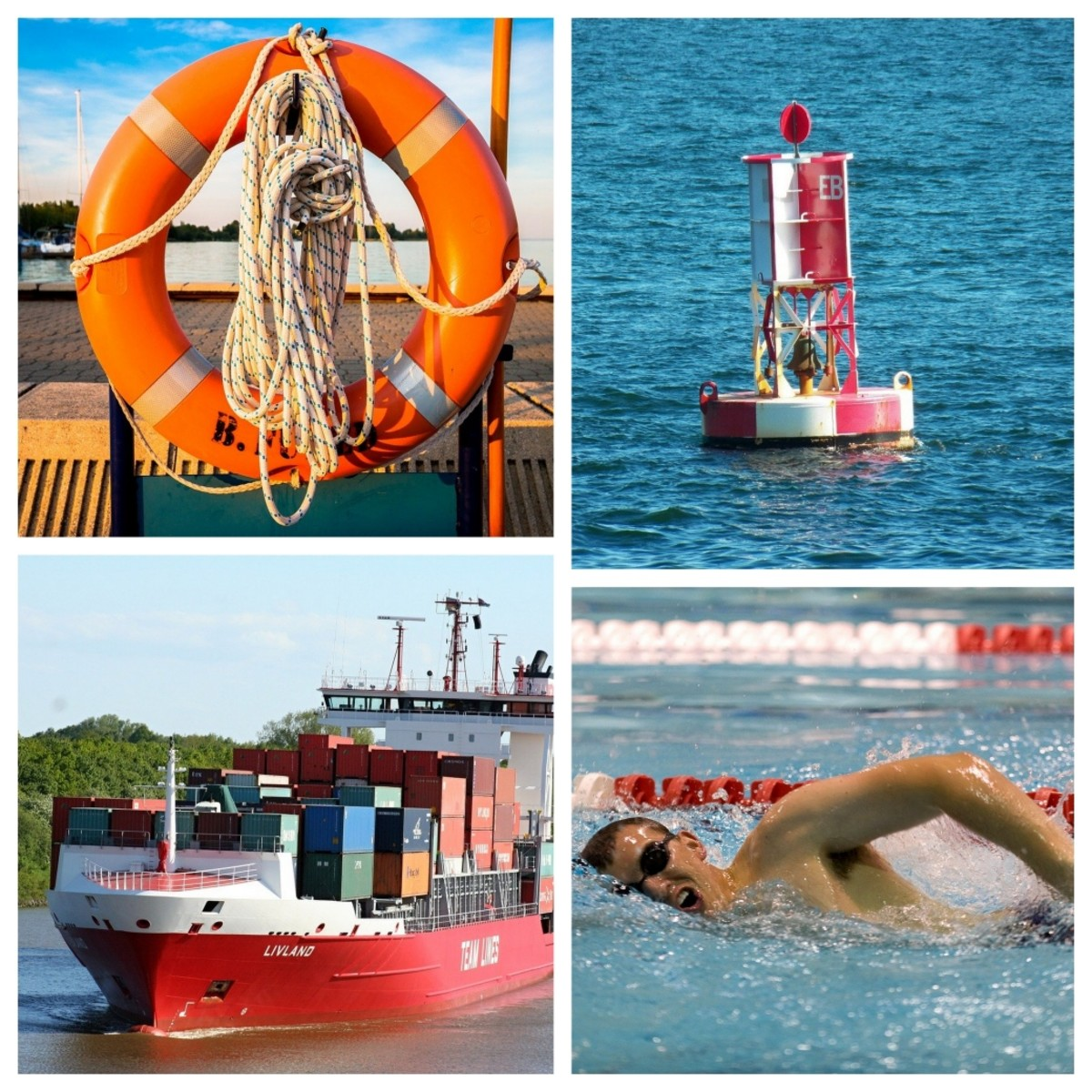Things that need to have positive buoyancy. Clockwise from the top: A life belt, marking buoy, swimmer, ship.Assorted images from Pixabay.com

## Experiment 3. Investigating Neutral Buoyancy

In this experiment, the object we use has neutral buoyancy and can stay suspended under the water surface without sinking down or being pushed back up by the buoyant force of the water.

Neutral buoyancy occurs when the average density of an object is the same as the density of the fluid it is immersed in. When the object is below the surface, it neither sinks nor floats. It can be positioned at any depth below the surface and will stay there until another force moves it to a new location.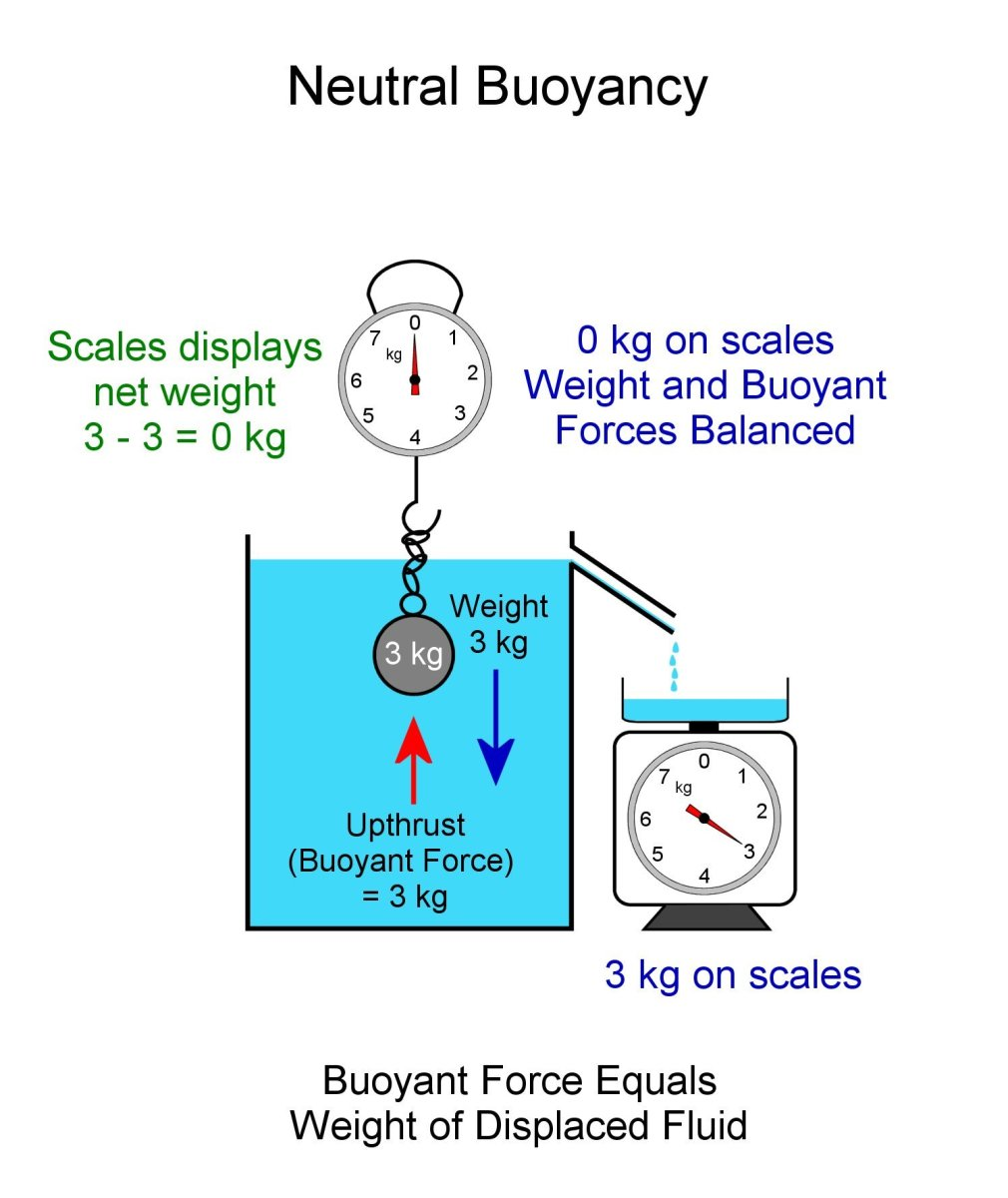Neutral Buoyancy. Body can be positioned anywhere under the surface. Buoyancy force and weight of ball are equal.© Eugene Brennan

## What are Examples of Things That Need Neutral Buoyancy?

• Diver
• Submarine

Submarines need to be able to control their buoyancy. So when there is a requirement to dive, large tanks are filled with water, producing negative buoyancy enabling them to sink. Once they reach the required depth, buoyancy is stabilised so that it becomes neutral. The sub can then cruise at a constant depth. When the sub needs to rise again, water is pumped out of the ballast tanks and replaced by air from compression tanks. This gives the submarine positive buoyancy, allowing it to float to the surface.

Humans naturally float in a vertical position with their noses just under the water if they relax their muscles. Scuba divers keep their buoyancy neutral by using belts with lead weights attached. This allows them to stay underwater at a desired depth without having to continually swim downwards.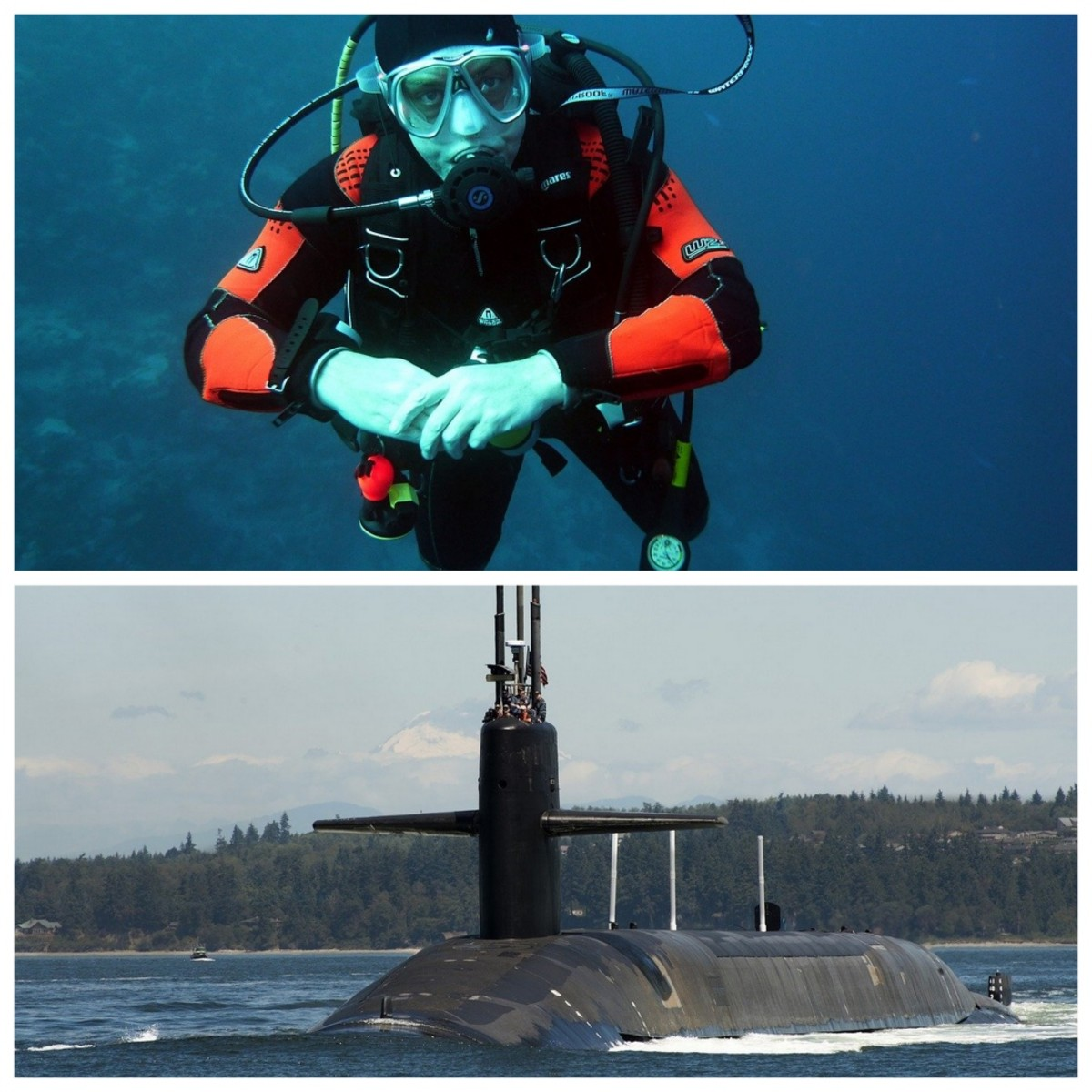A scuba diver needs to have neutral buoyancy. A submarine needs to have neutral, positive and negative buoyancy.Skeeze and Joakant. Public domain images via Pixabay.com

## Formula for the Buoyant Force

Where

ρ is the density of the displaced fluid

V is the volume of the displaced fluid

and g is the acceleration due to gravity

If we choose the the weight acting downwards to be a positive force, the negative sign in the equation for buoyant force is because it is a vector and acts in the opposite direction.

The weight of an object is

Fg = mg

where m is the mass of the object

## Why do Ships Float?

Ships weigh thousands of tons, so how come they can float? If a stone or a coin is dropped into water it will sink straight to the bottom.

The reason ships float is because they displace lots of water. Think of all the space inside a ship. When a ship is launched into water, it pushes all the water out of the way and the massive upthrust balances the downwards weight of the ship, allowing it to float.

## Why do Ships Sink?

Positive buoyancy keeps a ship afloat because the weight of the ship and buoyant force are balanced. However if too much heavy cargo is taken on by a ship, its total weight could exceed the buoyant force and it could sink. If the hull of a ship is holed, water will run into the hold. As water rises in the ship, it weighs down on the inside of the hull, causing the total weight to be greater than the buoyant force, making the ship sink.

A ship would also sink if we could magically crush all the steel structures and hull into a block. Because the block would take up a small fraction of the original volume of the ship, it wouldn't have the same displacement and therefore negative buoyancy.Ships float because they displace a huge amount of water and the buoyant force can support the weight of the ship.Susannp4, public domain image via Pixabay.com

## How Does Density of a Liquid Affect Buoyancy?

The density of the fluid an object is placed in affects buoyancy, however Archimedes' principle still applies.

### Average density of object

If m is the mass of an object and V is its volume, then the average density ρ of the object is:

ρ = m / V

An object may not be homogenous. This means that the density could vary throughout the volume of the object. For instance if we have a large, hollow steel ball, the density of the steel shell would be about 8000 times the density of the air inside it. The ball could weigh tons, however when we work out the average density using the equation above, if the diameter is large, the average density is much less than the density of a solid steel ball because the mass is a lot less. If the density is less than that of water, the ball will float when placed in water.

### Buoyancy and average density

• If the average density of an object is > density of the fluid, it will have negative buoyancy
• If the average density of an object is < density of the fluid, it will have positive buoyancy
• If the average density of an object = density of the fluid, it will have neutral buoyancy

Remember for an object to float, its average density must be lower than the density of the fluid it is placed in. So for instance if the density is less than water but greater than that of kerosene, it will float in water, but not in kerosene.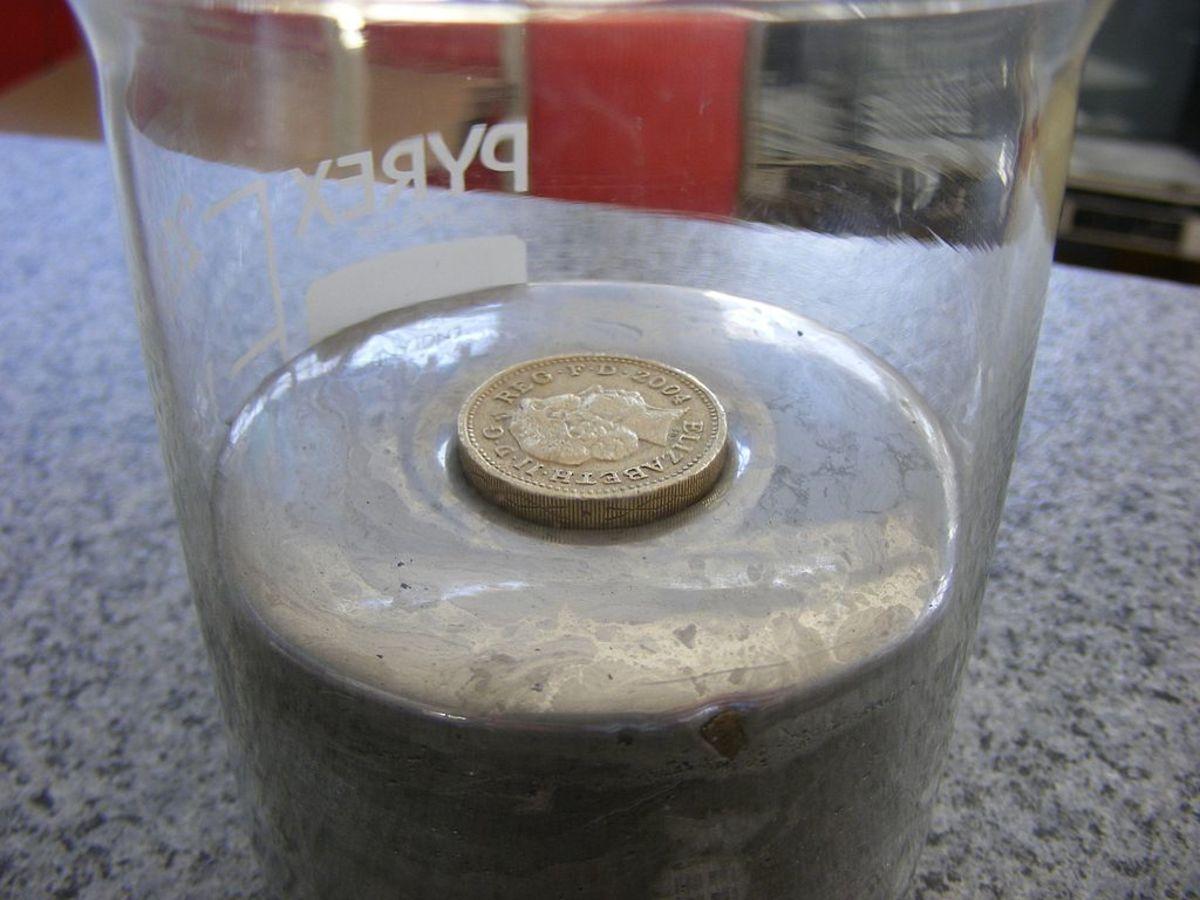A coin floats in mercury because the density of the metal that the coin is made from is less than that of mercury.Alby, CC BY-SA 3.0 via Wikimedia Commons

## How Do Helium Balloons Float?

The principle of Archimedes works for objects not just in a liquid like water, but other fluids also, like air. Just like an aeroplane, a balloon needs a force called lift to make it rise in the air. Balloons don't have wings to provide lift and instead use the buoyant force of displaced air.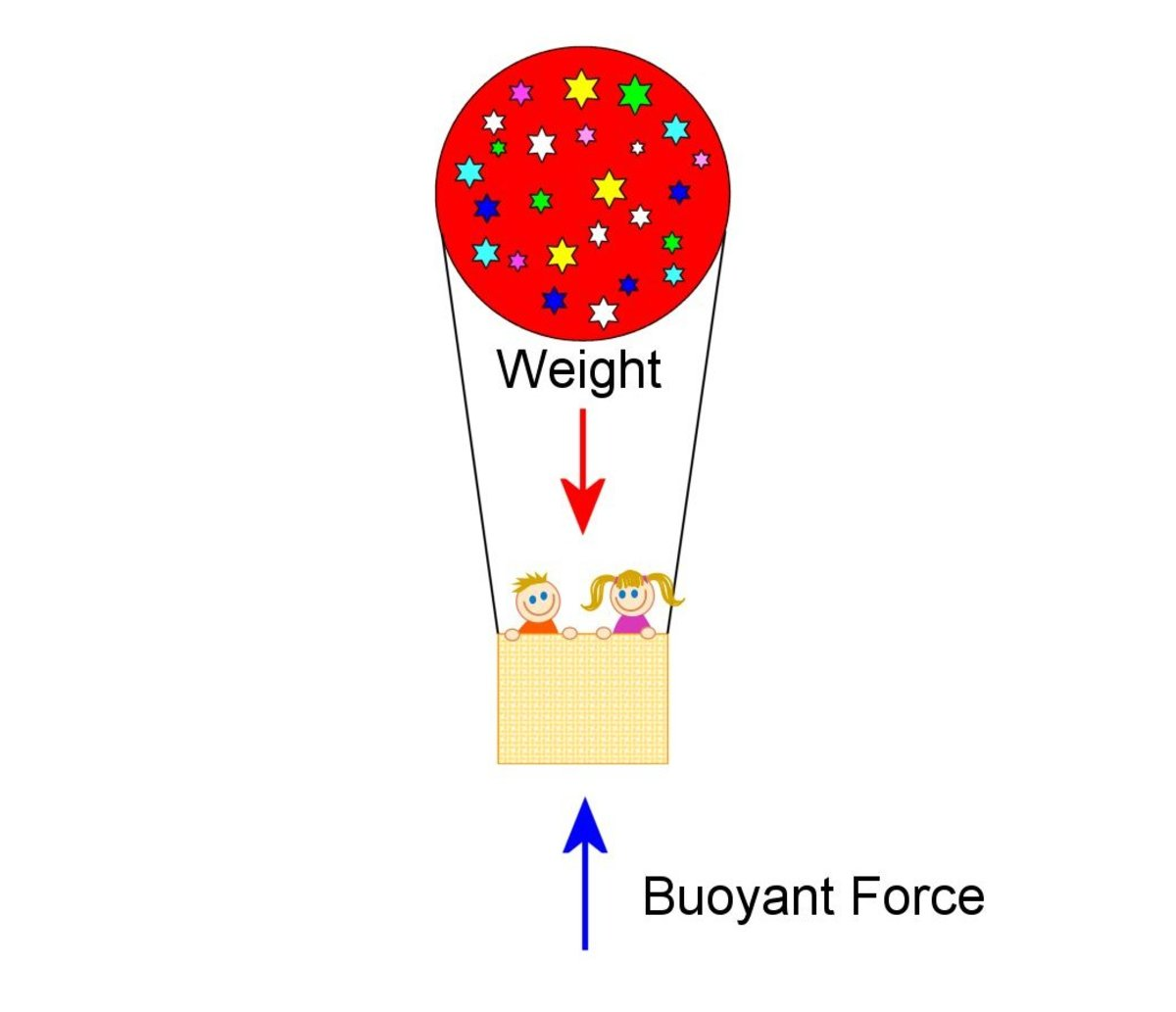Hot air and helium balloons rely on buoyancy to give them lift and keep them aloft.© Eugene Brennan

What gives a balloon lift to rise in the surrounding air?

Remember the Archimedes principle states that the upthrust or buoyant force is equal to the weight of the displaced fluid. In the case of a balloon, the displaced fluid is air.

First let's imagine a scenario where we have a large balloon and just fill it with air. The weight acting downwards consists of the weight of the balloon plus the weight of the air inside. However the buoyancy force is the weight of the displaced air (which is approximately the same as the weight of the air inside the balloon, because the displaced air has the same volume, neglecting the volume of the balloon material).

So the force acting downwards = weight of balloon + weight of air inside balloon

From Archimedes' Principle, the force acting upwards = weight of displaced air ≈ weight of air inside balloon

Net force acting downwards = (weight of balloon + weight of air inside balloon) - weight of air inside balloon = weight of balloon

Therefore the balloon will sink.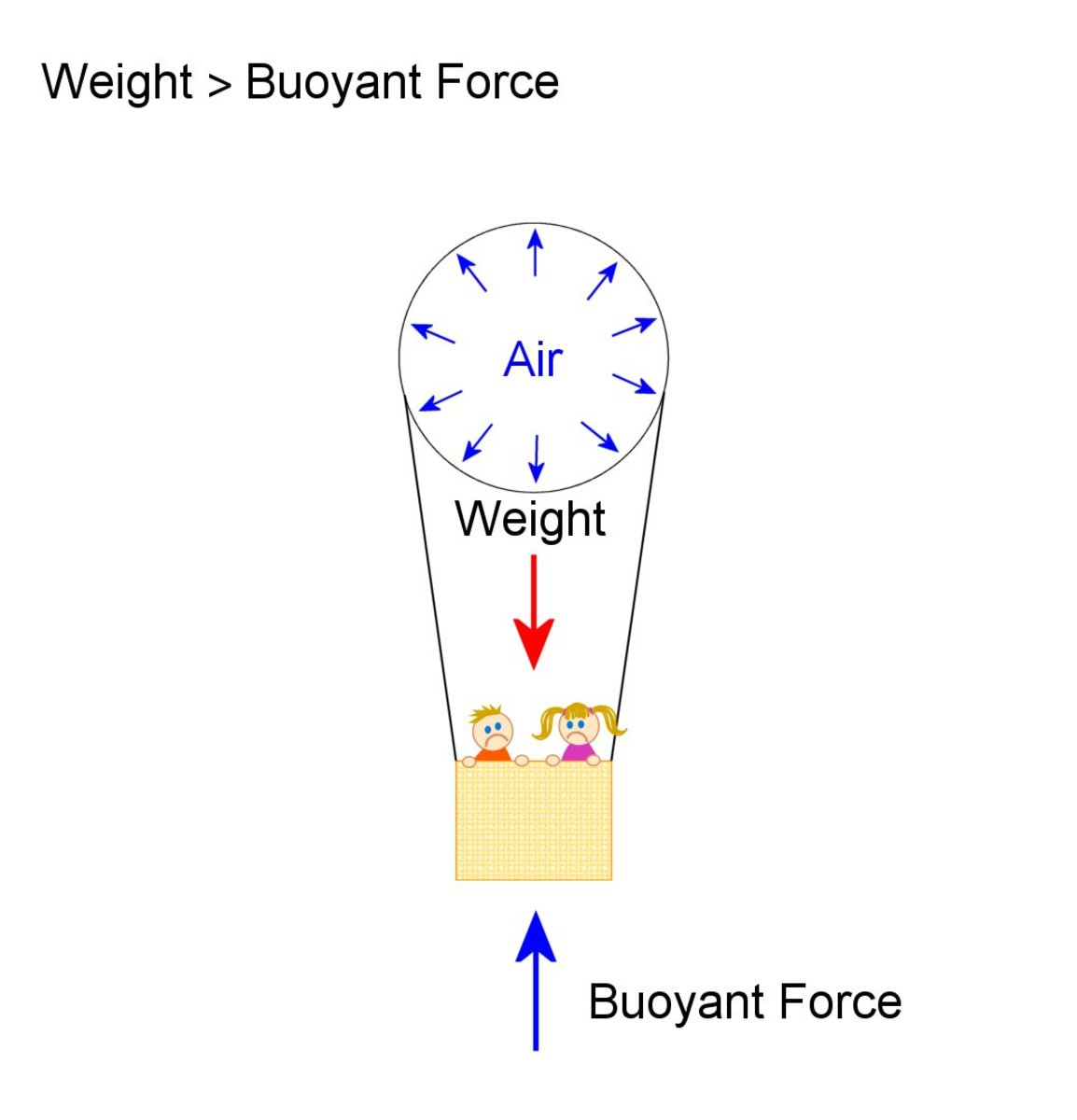Weight of balloon and air inside (and also the basket and people, ropes etc) is greater than the buoyant force which is the weight of displaced air, so it sinks.© Eugene Brennan

Now imagine we make the balloon large so that it has a lot of space inside.

Let's make it a sphere 10 metres in diameter and fill it with helium. Helium has a density less than that of air.

The volume is approximately 524 cubic metres.

This much helium weighs about 94 kilos.

The balloon displaces 524 cubic metres of air, however air is nearly six times denser than helium, so that air weighs about 642 kg.

So from Archimedes principle, we know that the upthrust equals this weight. The upthrust of 642 kg acting upwards on the balloon is greater than the weight of the helium inside the balloon and this gives it lift.

In practice the weight acting downwards would be greater because of the weight of the skin of the balloon, basket, people etc.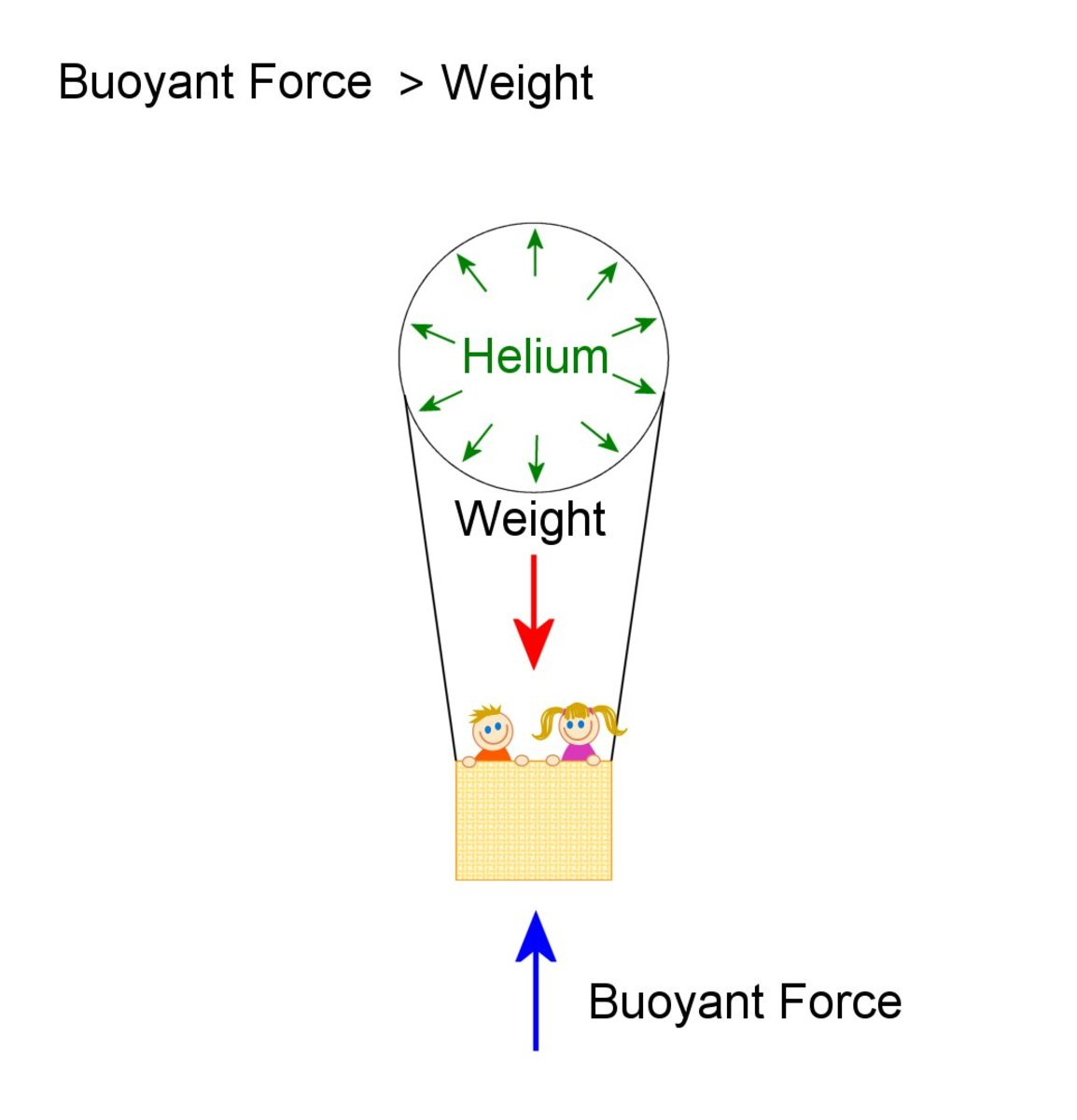Weight of balloon and helium inside it is less than weight of displaced air, so the buoyant force gives enough lift to make it rise.© Eugene Brennan

## Why Do Hot Air Balloons Float ?

Helium balloons float because they're filled with helium which is less dense than air. Hot air balloons have tanks of propane and burners on board in the basket. Propane is the gas used for camping stoves and outdoor cooking grills. When the gas is burned, it heats the air. This rises upwards and fills the balloon, displacing the air inside. Because the air inside the balloon is hotter than the ambient temperature of the air outside, it's less dense and weighs less. So the air displaced by the balloon is heavier than the air inside it. Since the upthrust force equals the weight of the displaced air, this exceeds the weight of the balloon and the less dense hot air inside it and this lift force causes the balloon to rise.A hot air balloon.Stux, oublic domain image via Pixabay.com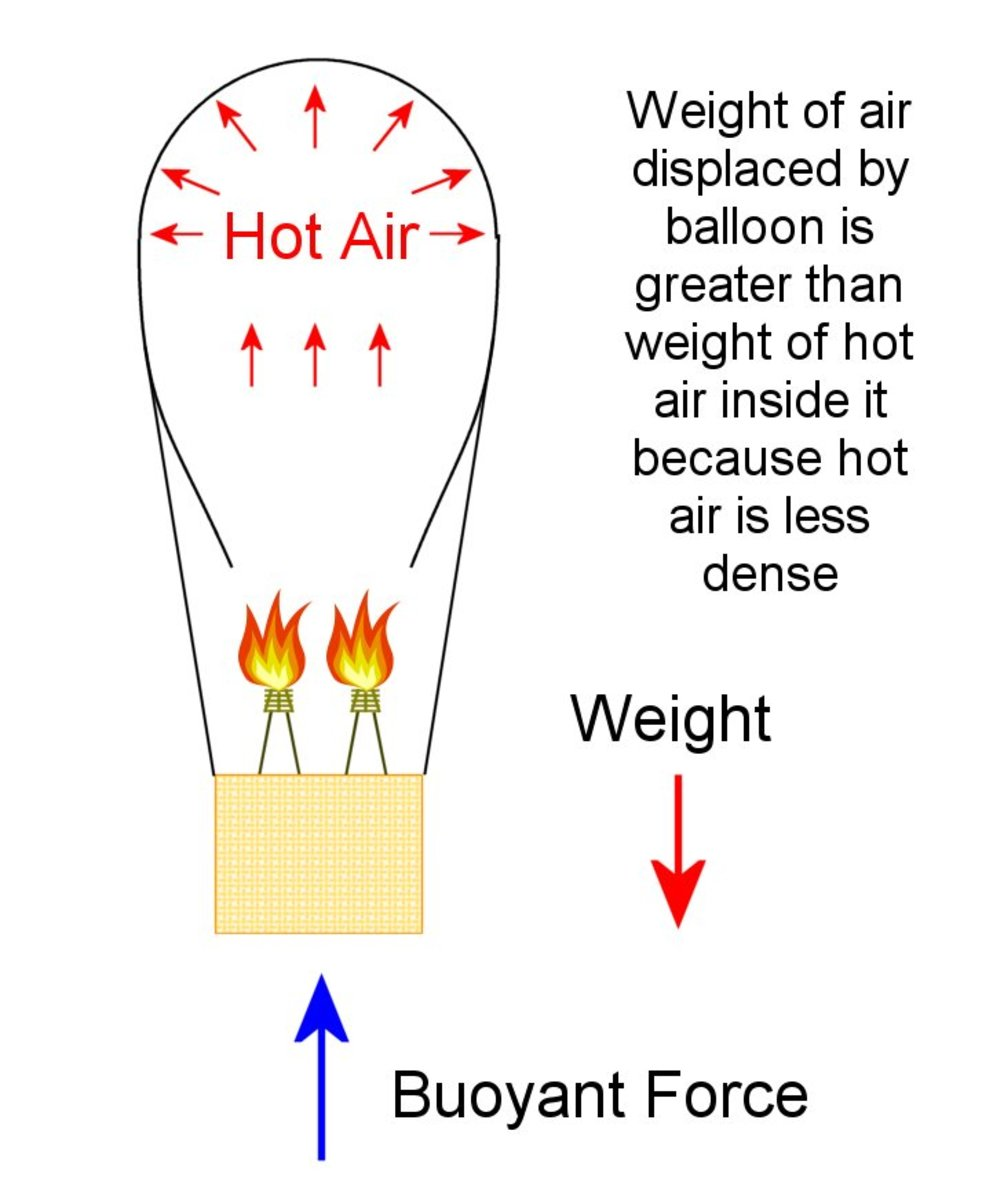The weight of displaced air (which produces the buoyant force) is greater than the weight of the balloon's skin, basket, burners and less dense hot air inside it and this gives it enough lift to rise.© Eugene Brennan

## Worked Examples on Buoyancy

### Question 1:

A hollow steel ball weighing 10 kg and diameter 30 cm is pushed below the surface of water in a pool.
Calculate the net force pushing the ball back to the surface.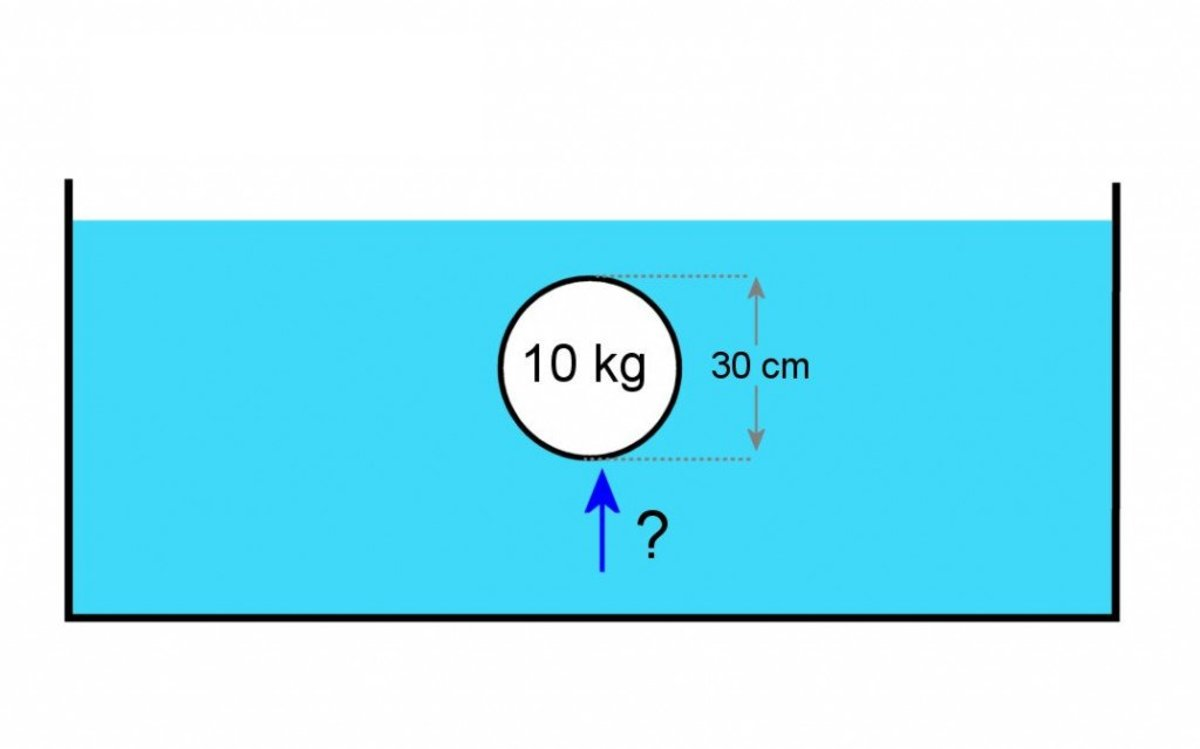Calculate the buoyant force on a steel ball submerged in water.© Eugene Brennan

We need to calculate the volume of water displaced. Then knowing the density of water, we can work out the weight of water and thus the buoyant force.

Volume of a sphere V = 4/3 π r3

r is the radius of of the sphere

π = 3.1416 approx

We know the diameter of the sphere is 30 cm = 30 x 10-2 m

so r = 15 x 10-2 m

Substituting for r and π gives us

V = 4/3 x 3.1416 x (15 x 10-2)3

Now work out the mass of water displaced by this volume.

ρ = m / V

where ρ is the density of a material, m is its mass and V is the volume.

Rearranging

m = ρV

for pure water ρ = 1000 kg / m3

Substituting for ρ and V calculated previously gives us the mass m

m = ρV = 1000 x 4/3 x 3.1416 x (15 x 10-2)3

= 14.137 kg approx

So the ball weighs 10 kg, but the displaced water weighs 14.137 kg. This results in a buoyant force of 14.137 kg acting upwards.

The net force pushing the ball to the surface is 14.137 - 10 = 4.137 kg

The ball has positive buoyancy, so it will rise to the surface and float, stabilizing with enough of its volume submerged to displace 10kg of water to balance its own 10kg weight.

Note: Strictly speaking force is measured in Newtons. If m is mass and g is the acceleration due to gravity = 9.81 approx, then force F = mg. A weight of 1 kg is equivalent to approximately 1 x g = 1 x 9.81 = 9.81 N
See my guide on mechanics for more info:

### Question 2:

How many balloons would it take to lift a person if the balloons were filled with helium? Each balloon is spherical, 30 cm diameter and weighs 10g (including string). The person weighs 90 kg.
Assume that the thickness of the skin of the balloon is small compared to the diameter, so the volume of helium internally = volume of air displaced.How many helium balloons does it take to lift a person?© Eugene Brennan

First assign variables and convert to standard SI units.

Let d be the diameter of a balloon = 30 cm = 30 x 10-2 m

so r the radius of a balloon = 15 x 10-2 m

Let N be the number of balloons.

Let ρh be the density of helium = 0.1786 g / L = 1.786 x 10-1 kg/m3

Let ρa be the density of air = 1.225 kg/m3

Let V be the volume of a balloon

Let w be the weight of each balloon = 10 g = 10 x 10-3 kg

Let Wp be the weight of the person = 90 kg

The volume of each balloon is V = 4/3πr3

Total volume of all the balloons = NV

Total weight of helium = volume of balloons x density of helium = NVρh

Total weight of air displaced = buoyancy force = volume of balloons x density of air = NVρa

Total weight of balloons = Nw

Buoyancy force = weight of air displaced and this must be greater than the weight of the helium + the weight of balloons + person's weight.

Volume of a balloon V = 4/3πr3 = 4/3 x 3.1416 x (15 x 10-2)3 = 1.414 x 10-2 m3

So write the equation:

NVρa > NVρh + Nw + Wp

Rearrange giving N(Vρa - Vρh - w) > Wp

So N > Wp / (Vρa - Vρh - w)

Plug in the numbers:

N > 90 / (1.414 x 10-2 x 1.225 - 1.414 x 10-2 x 1.786 x 10-1 - 10 x 10-3) = 18,765

That's a lot of balloons and the problem is that as the number of the balloons increases, the helium also has to lift the weight of the material they're made of. A better solution is to use bigger balloons. If we increase the diameter, volume increases with the cube of the radius while surface area only increases with the square of the radius. So there's more volume of helium per weight of balloon and less balloons are needed. Imagine we increase the size of the balloons by a factor of ten from 30 cm to 3 m.

Surface area = 4πr2

If r is now 10 times greater, the r squared factor results in a 100 times increase in surface area (assuming the material is stretched by the same amount on inflation.) So weight increases 100 times and a balloon now weighs 100 x 10 g = 1 kg.

However volume is proportional to r3 so if r increases ten times, volume increases 1000 times, but balloon weight has only increased 100 times. The bottom line is that a bigger balloon has greater net lifting force, not just because of the bigger dimensions, but because balloon weight/volume becomes smaller.

The volume of a balloon is now:

V = 4/3πr3 = 4/3 x 3.1416 x (1.5 )3 = 14.137 m3

Plug in the numbers again:

N > 90 / (14.137 x 1.225 - 14.137x 1.786 x 10-1 - 1) = 6.5

That's a bit better, so only 7 balloons needed this time.

This content is accurate and true to the best of the author’s knowledge and is not meant to substitute for formal and individualized advice from a qualified professional.

© 2019 Eugene Brennan